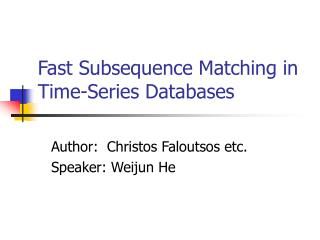DownloadDownload PresentationFast Subsequence Matching in Time-Series Databases

# Fast Subsequence Matching in Time-Series Databases

Télécharger la présentation## Fast Subsequence Matching in Time-Series Databases

- - - - - - - - - - - - - - - - - - - - - - - - - - - E N D - - - - - - - - - - - - - - - - - - - - - - - - - - -
##### Presentation Transcript

1. Fast Subsequence Matching in Time-Series Databases Author: Christos Faloutsos etc. Speaker: Weijun He

2. What is the problem? • What is Time Series: 1-dimensional data e.g. Daily stock market price, Daily temperature, etc • Our goal: Design fast searching methods that will locate subsequence that match a query subsequence, exactly or approximately

3. Motivation/Application • Financial, marketing, production Typical query: ‘find companies whose stock prices move similarly’ • Scientific databases Typical query: ‘find past days in which solar magnetic wind showed similar patterns as today’s’

4. Some notational conventions If S and Q are two sequences, then: • Len(S) : length of S • S[i:j] : subsequence including i and j • S[i] : i-th entry of S • D(S,Q) : distance of two equal length sequence S and Q

5. Queries Two categories for queries: • Whole Mathing: len(data) = len(query) • Subsequence Matching: len(data) > len(query) Remark: • The distance function D(S,Q) is defined, e.g. D() can be the Euclidean distance • Matching means: D(S,Q) < , i.e., approximately

6. Whole Matching • Any distance-preserving transform(e.g., Discrete Fourier Transform(DFT),extract f features from sequences(e.g., the first f DFT coefficients): f-dimensional feature space • Any spatial access method(e.g., R*-tree) can be used for range/approximate queries

7. Mathematical Background Lemma 1 To guarantee no false dismissals for range queries, the feature extraction function F() should satisfy the following formula: Dfeature(F(O1),F(O2))<=Dobject(O1,O2) False dismissal: discard the qualified sequence, BAD False alarm: non-qualified sequence not discarded, Not so bad

8. Discrete Fourier Transform Theorem(Parseval): i=0,..,n-1Xi2 = f=0,..,n-1Xf2 (distance preserving) DFT is a linear transform, so it can be proved that DFT satisfy Lemma 1. We Keep the first few(2-3) coefficients as features Properties: 1. Only false alarm, no false dismissal 2. Practically, false alarms are few

9. From Whole to Subsequence matching Question: How to generalize the method to approximate match queries for subsequences of arbitrary length?

10. Subsequence Matching:Criterion Some criterion: • Fast: sequential scanning and distance calculation at each and every possible offset is too slow for large databases • Correct: No ‘false dismissals’, but ‘false alarms’ are acceptable • Small space overhead • Dynamic • Varying lengthfor data and query sequences

11. Proposed Method • Using Sliding window of w, minimum query length. A data sequence of length Len(S) is mapped to a trail in feature space, consisting of len(S)-w+1 points. —”Sub-Trail-index”

12. I-naïve method The straightforward way is • keep track of the individual points of each trail, storing them in spatial access method Disadvantage: Inefficient since almost every point in a data sequence will correspond to a point in the f-dimensional feature space.

13. I-naïve method – Contd. How to improve: Observation: the content of the sliding window in nearby offset will be similar. Solution: Divide the trail into sub-trails and represent each of them with its Minimum Bounding Rectangle (MBR), thus we only need to store a few MBRs, “no false dismissals” are guaranteed.

14. Illustration

15. MBR Property • Each MBR corresponds to a whole sub-trail, i.e., points in feature space that correspond to successive positions of the sliding window. • Each leaf-MBR has tstart, tend which are the offsets of the first and last such positions, also has a unique identifier for the data sequence (sequence_id) • The extent of the MBR in each dimension is denoted as: (F1low,F1high, F2low,F2high,……) • MBR are stored in R* tree.

16. Figure2: Structure of a leaf node and a non-leaf nodeindex node layout for the last two levels

17. ST-index There are two questions for ST-index: • Insertion (Dynamic requirement): when new data sequence is inserted, what is a good way to divide its trail into sub-trail? • Queries longer than w: how to handle queries, especially the ones longer than w.

18. ST-index: Insertion

19. Illustration

20. I-adaptive heuristic Cost function: DA(L)=П(Li+0.5) where L=(L1,L2,..Ln), 1<=i<=n. Marginal cost of a point: Consider a sub-trail of K points with a MBR of sizes L1,…Ln, each point in this sub-trail has : mc=DA(L) /k

21. I-adaptive heuristic: algorithm /* Algorithm Divide-to-Subtrails */ Assign the first point of the trail in a (trivial) sub-trail FOR each successive point IF it increase the marginal cost of the current sub-trail THEN start another sub-trail ELSE include it in the current sub-trail

22. Searching-Queries longer than W Two methods: • PrefixSearch • select the prefix of Q of length w, match the prefix within tolerance e • MultiPiece Search • Suppose the query sequence has length p*w, • Break Q into p sub-queries which correspond to p sphere in feature space with raius e/sqrt(p); • Use “ST-index” to retrieve the sub-trails whose MBRs intersect at least one of the sub-query region.

23. Prefix vs. MultiPiece search Volume required in feature space(K is a constant): • Prefixsearch: K e^f • Multipiece: K*p*(e/sqrt(p))^f Multipiece is likely to produce fewer false alarms

24. Conclusions The main contribution is: “I-adaptive” method: • achieves orders of magnitude savings over the sequential scanning. • Small space overhead • It is dynamic • No false dismissal Future work: Extend this method for 2-dimensional gray scale images, and in general for n-dimensional vector-fields(e.g. 3-d MRI brain scans)

25. The End Thank you for your attention!